# Fundamental theorem of calculus

The fundamental theorem of calculus is a theorem that links the concept of differentiating a function (calculating its slopes, or rate of change at each time) with the concept of integrating a function (calculating the area under its graph, or the cumulative effect of small contributions). The two operations are inverses of each other apart from a constant value which depends on where one starts to compute area. The first part of the theorem, the first fundamental theorem of calculus, states that for a function f , an antiderivative or indefinite integral F may be obtained as the integral of f over an interval with a variable upper bound. This implies the existence of antiderivatives for continuous functions. Conversely, the second part of the theorem, the second fundamental theorem of calculus, states that the integral of a function f over a fixed interval is equal to the change of any antiderivative F between the ends of the interval. This greatly simplifies the calculation of a definite integral provided an antiderivative can be found by symbolic integration, thus avoiding numerical integration. (Wikipedia).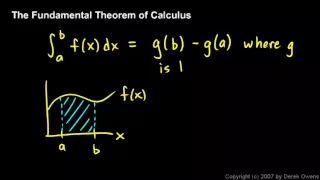Calculus - The Fundamental Theorem, Part 1

The Fundamental Theorem of Calculus. First video in a short series on the topic. The theorem is stated and two simple examples are worked.

From playlist Calculus - The Fundamental Theorem of Calculus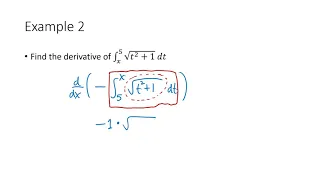Calculus: The Fundamental Theorem of Calculus

This is the second of two videos discussing Section 5.3 from Briggs/Cochran Calculus. In this section, I discuss both parts of the Fundamental Theorem of Calculus. I briefly discuss why the theorem is true, and work through several examples applying the theorem.

From playlist Calculus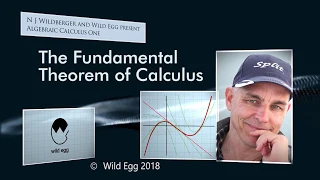The Fundamental Theorem of Calculus | Algebraic Calculus One | Wild Egg

In this video we lay out the Fundamental Theorem of Calculus --from the point of view of the Algebraic Calculus. This key result, presented here for the very first time (!), shows how to generalize the Fundamental Formula of the Calculus which we presented a few videos ago, incorporating t

From playlist Algebraic Calculus One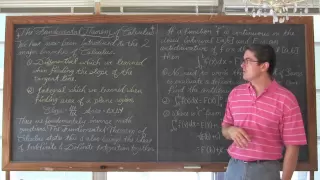First Fundamental Theorem of Calculus Calculus 1 AB

I introduce and define the First Fundamental Theorem of Calculus. I finish by working through 4 examples involving Polynomials, Quotients, Radicals, Absolute Value Function, and Trigonometric Functions. Check out http://www.ProfRobBob.com, there you will find my lessons organized by clas

From playlist Calculus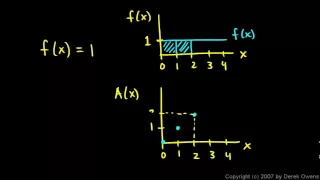Calculus - The Fundamental Theorem, Part 3

The Fundamental Theorem of Calculus. Specific examples of simple functions, and how the antiderivative of these functions relates to the area under the graph.

From playlist Calculus - The Fundamental Theorem of Calculus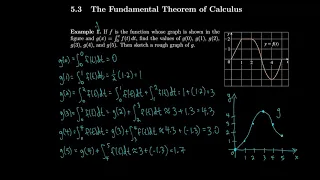Calculus 5.3 The Fundamental Theorem of Calculus

My notes are available at http://asherbroberts.com/ (so you can write along with me). Calculus: Early Transcendentals 8th Edition by James Stewart

From playlist Calculus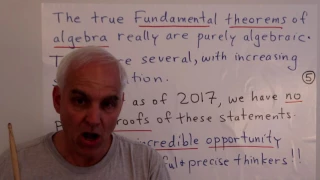What is the Fundamental theorem of Algebra, really? | Abstract Algebra Math Foundations 217

Here we give restatements of the Fundamental theorems of Algebra (I) and (II) that we critiqued in our last video, so that they are now at least meaningful and correct statements, at least to the best of our knowledge. The key is to abstain from any prior assumptions about our understandin

From playlist Math Foundations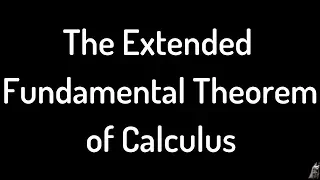Extended Fundamental Theorem of Calculus

Please Subscribe here, thank you!!! https://goo.gl/JQ8Nys Extended Fundamental Theorem of Calculus. You can use this instead of the First Fundamental Theorem of Calculus and the Second Fundamental Theorem of Calculus. - Formula - Proof sketch of the formula - Six Examples

From playlist Calculus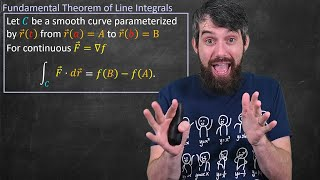The Fundamental Theorem of Line Integrals // Big Idea & Proof // Vector Calculus

Back in 1st year calculus we have seen the Fundamental Theorem of Calculus II, which loosely said that integrating the derivative of a function just gave the difference of the function at the endpoints. That is, what happened in the middle did not matter. In this video we upgrade to the FuThe Proof of TAYLOR'S THEOREM Ms. Michael Never Taught You

In this video, we look at a very elementary proof of Taylor's theorem that I stumbled upon while going for a walk the other week. The proof uses only the fundamental theorem of calculus is such an easy proof that we could've all discovered it if we had gone on enough walks. Timestamps: 0:

From playlist Calculus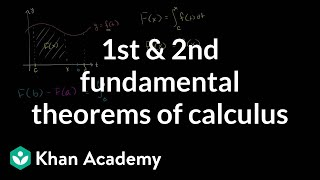Fundamental theorem of calculus (Part 2) | AP Calculus AB | Khan Academy

There are really two versions of the fundamental theorem of calculus, and we go through the connection here. Created by Sal Khan. Practice this lesson yourself on KhanAcademy.org right now: https://www.khanacademy.org/math/ap-calculus-ab/ab-antiderivatives-ftc/ab-fundamental-theorem-of-ca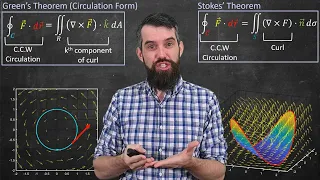A unified view of Vector Calculus (Stoke's Theorem, Divergence Theorem & Green's Theorem)

In the final video of my vector calculus playlist (congrats to everyone for making it to the end!!!) I want to do a bit of an overview of the major theorems we have seen in this course - Stokes' Theorem, Divergence Theorem, Green's Theorem - all are part of a unified framework. Loosely, in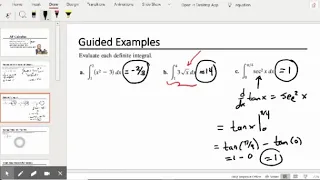Integral Applications and Techniques

1. Review Fundamental Theorem of Calculus 2. Review Average Value of a Function 3. Review Mean Value Theorem for Integrals 4. Definite Integral as a Function 5. Second Fundamental Theorem of Calculus

From playlist Calculus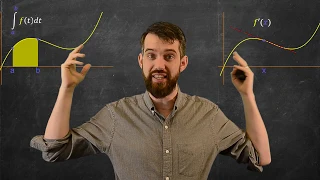Fundamental Theorem of Calculus 1 | Geometric Idea + Chain Rule Example

Derivatives are geometrically tangents to curves while definite integrals area areas under curves. How are these related? The Fundamental Theorem of Calculus parts I and II show how we can compare these two fundamental concepts in Calculus. The first derivative, the subject of this video,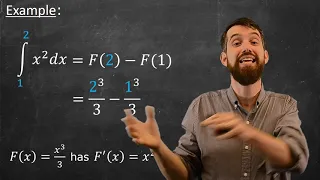Fundamental Theorem of Calculus II

This video looks at the second fundamental theorem of calculus, where we take the definite integral of a function whose anti-derivative we can compute. This gives a short and sweat way to compute any definite integral provided we can come up with an anti-derivative. *********************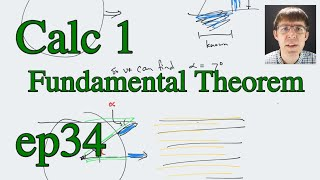Calculus 1 (Stewart) Ep 34, Fundamental Theorem of Calculus (Dec 3, 2021)

This is a recording of a live class for Math 1171, Calculus 1, an undergraduate course for math majors (and others) at Fairfield University, Fall 2021. The textbook is Stewart. PDF of the written notes, and a list of all episodes is at the class website. Class website: http://cstaecker.f

From playlist Math 1171 (Calculus 1) Fall 2021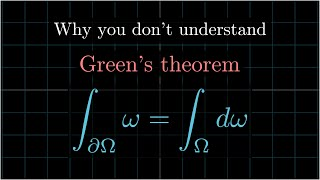Why you don't understand GREEN'S THEOREM -- Geometric Algebra, Calculus 3, Vector Calculus

In this video, we discuss the link between the fundamental theorem of calculus and Green's theorem. This offers an introduction to the exterior algebra, specifically, the wedge product. We discuss, albeit not explicitly, the de Rham pairing, which offers an enlightening interpretation of t

From playlist Linear Algebra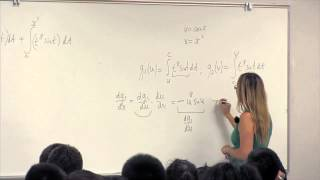Math 2B. Calculus. Lecture 04. The Fundamental Theorem of Calculus.

UCI Math 2B: Single-Variable Calculus (Fall 2013) Lec 04. Single-Variable Calculus -- The Fundamental Theorem of Calculus -- View the complete course: http://ocw.uci.edu/courses/math_2b_calculus.html Instructor: Natalia L. Komarova Ph.D. License: Creative Commons CC-BY-SA Terms of Use: ht

From playlist Math 2B: Calculus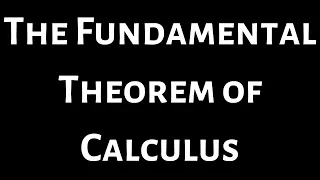The Fundamental Theorem of Calculus and How to Use it

Please Subscribe here, thank you!!! https://goo.gl/JQ8Nys The Fundamental Theorem of Calculus and How to Use it

From playlist Calculus 1